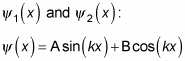##### Quantum Physics For DummiesInfinite square well, in which the walls go to infinity, is a favorite problem in quantum physics. To solve for the wave function of a particle trapped in an infinite square well, you can simply solve the Schrödinger equation.

Take a look at the infinite square well in the figure.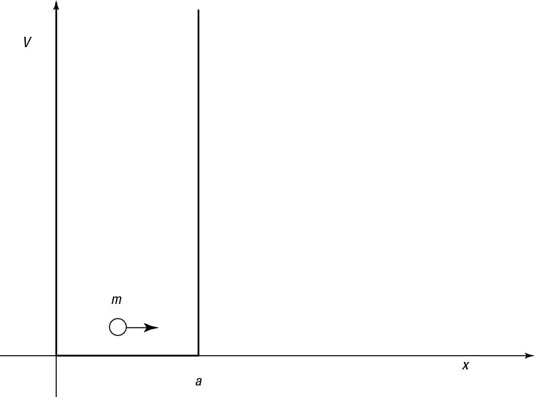A square well.

Here’s what that square well looks like: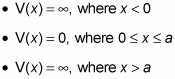The Schrödinger equation looks like this in three dimensions: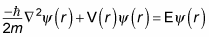Writing out the Schrödinger equation gives you the following: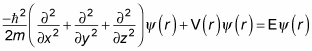You’re interested in only one dimension — x (distance) — in this instance, so the Schrödinger equation looks like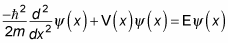Because V(x) = 0 inside the well, the equation becomes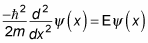And in problems of this sort, the equation is usually written as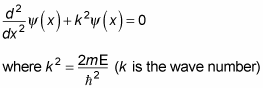So now you have a second-order differential equation to solve for the wave function of a particle trapped in an infinite square well.

You get two independent solutions because this equation is a second-order differential equation: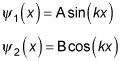A and B are constants that are yet to be determined.

The general solution of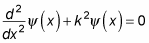is the sum of